# Inequalities with fractions

## Summary and examples

Roughly speaking, inequalities with fractions are inequalities with one or more fractions in which the unknown variable (e.g.) occurs in the denominator or in both the numerator and denominator.
First study the topic Equations with fractions before continuing this topic about inequalities.
The following examples show how you can solve these types of inequalities.

##### Example 1

Solve: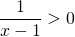This is a simple example. The numerator in the left-hand side is always positive, then the fraction will be positive if the denominator is positive as well.

So the fraction is greater than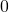if:so if: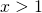##### Example 2

Solve: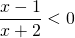The solution of this inequality is a bit more difficult to find. The fraction is less thanfor those values offor which the numerator is positive and the denominator is negative, or vice versa. But we have to do more.
A convenient approach is the following. For each factor in both the numerator and denominator draw a so-called number line. For each factor find the values offor which it is positive, equal toor negative.

In this case we do this for the factors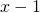and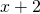.----------------------------(1)+++++++---------------(-2) ++++++++++++++

quotiënt ++++++++(-2) ---------(1)+++++++

The first number line shows that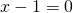for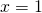,forand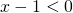for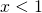; the second number line shows that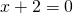for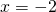,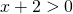for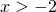and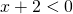for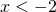.

The third line is the result of the other two. We are dealing with fractions and therefore we look at the results of divisions in certain intervals: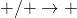,,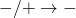and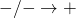. In this case we get: forthe fraction is positive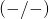; for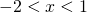the fraction is negativeand forthe fraction is positive again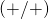.
This method is quite simple and can also be applied if more factors are involved as we can see in next example.
The inequality above is satisfied for.

##### Example 3

Solve: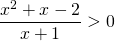We notice that the numerator can be written as follows: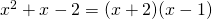and thus we have to solve: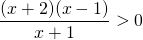Again we draw a number line for each factor. Make sure that the numbers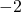,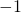,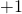have the right positions.

x+2 ----------(-2)++++++++++++++++++

x-1 ------------------------------------(1)+++++

x+1 ---------------------(-1)+++++++++++++

result --------(-2)+++(-1)----------(1)++++++

The inequality is satisfied for: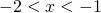or##### Example 4

Solve: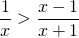This example is somewhat more complicated because fractions have to be added or subtracted before we get the right form of inequality.
We move the fractions to the left-hand side: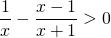We can subtract the fractions after having made the denominators equal: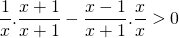Now we can write the inequality as:or: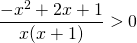or: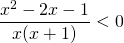The numerator can be factorized: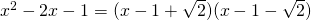by applying the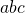-formula.
As a result we have to solve the following inequality: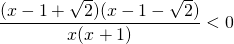We draw number lines for each of the four factors (-0.4 and 2.4 are approximations):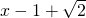----------(-0.4)++++++++++++++++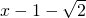------------------------------------------(2.4)+++--------------------------------------(0)+++++++++++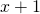-----------(-1)++++++++++++++++++++++

result        +++++++(-1)------(-0.4)++++(0)--------------(2.4)+++

This inequality is satisfied if: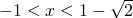or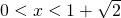We mentioned before that cross-multiplication yields incorrect solutions. We will show this below.

Cross-multiplication would give: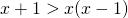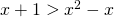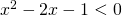We can immediately see that this is not the correct answer.

0